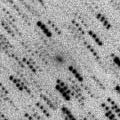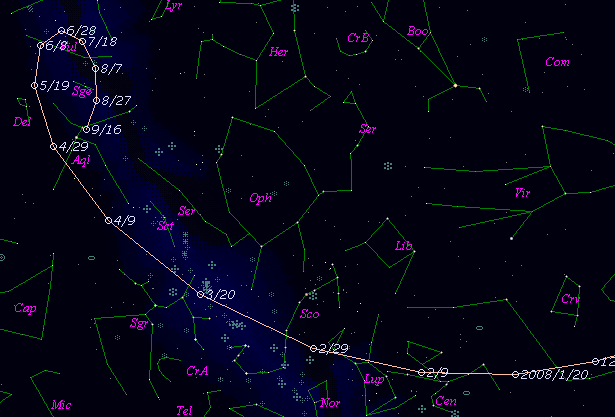# 26P/Grigg-Skjellerup (2008)###Pictures###Orbital Elements

```The following orbital elements are taken from MPC 54168:

Epoch 2008 Apr. 4.0 TT = JDT 2454560.5
T 2008 Mar. 23.6923 TT                                  Muraoka
q   1.116716             (2000.0)            P               Q
n   0.1856897      Peri.    1.7152      -0.8358246      +0.5113060
a   3.042828       Node   211.7062      -0.5081148      -0.8583596
e   0.633001       Incl.   22.3566      -0.2078861      +0.0422493
P   5.31
From 173 observations 1961-1992, mean residual 0".9.  Nongravitational
parameters A1 = +0.00, A2 = -0.0011.
```

###Finding Charts###Magnitudes Graph

```        m1 = 16.2 + 5 log d +  10.0 log r(t - 20)  [   ,-20]  (             - 2008 Mar.  3)
m1 =  8.0 + 5 log d + 100   log r(t - 20)  [-20, 60]  (2008 Mar.  3 - 2008 May  22)
m1 = 16.2 + 5 log d +  10.0 log r(t - 20)  [ 60,   ]  (2008 May  22 -             )

* Gray curve is:  m1 = 16.2 + 5 log d + 10.0 log r(t - 20)
```##### The orbital elements are published at the MPC Ephemerides and Orbital Elements. The charts are made with StellaNavigator Ver.2.0 for Windows (AstroArts / ASCII). The magnitudes graphs are made with Comet for Windows.